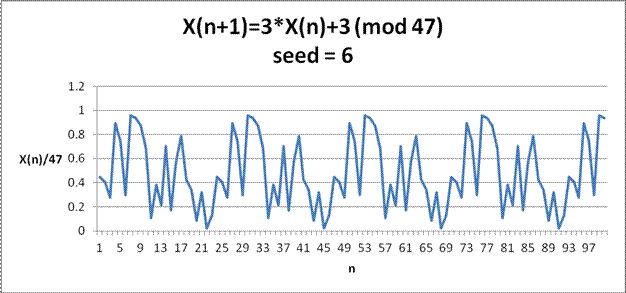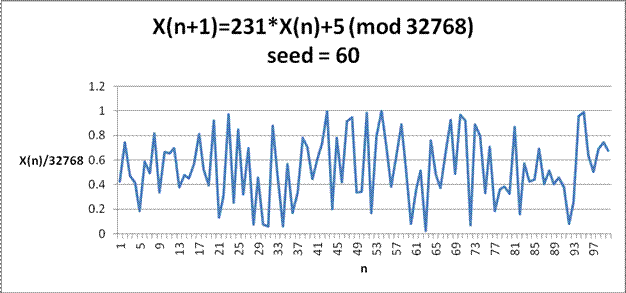# Random Numbers

3/9/11

The statistical community uses random numbers for sampling, analysis, simulation, imputation, and many other uses. In fact, their use is ubiquitous not only in statistical work, but scientific work the world over. But what does one mean by "random" and where do random numbers come from?

True random numbers are hard to come by. The random numbers generated in SAS or Excel are actually "computer generated random numbers" or "pseudo-random numbers". These are numbers that are generated from an algorithm (PRNG) whose output passes various statistical tests for randomness. That is, the output appears random and for all intents and purposes is random.

These PRNGs work off of a "seed" and will produce random numbers in part based on this seed. A common choice for a seed is the computer's date/time the moment of running the algorithm. This will produce different random numbers with each run because the date/time would be changing. Other times the seed is kept a constant which will produce the same random numbers each time. Why would one want to do this? Keeping the seed constant would enable you to check your work or for others to duplicate your results.

The following SAS code illustrates the differences. Run each section of code several times to see how the output changes (or does not change) the datasets.

seed is a constant, same results each time
data temp1;
do i=1 to 10;
x=ranuni(123456789);
output;
end;
run;

seed is system clock, denoted by 0, different results each time
data temp2;
do i=1 to 10;
x=ranuni(0);
output;
end;
run;

There are many types of PRNGs, ranging from extremely simple to extremely complex, and have varying properties. A common PRNG is what is called a linear congruential generator, created in 1951 by Lehmer. It uses three integers a, b, and m. There are some restrictions and sensible choices of these variables the practitioner needs to keep in mind.

Given an initial seed number x, the generator computes ax+b (mod m), that is, the remainder when the value ax+b is divided by m. The calculation is then repeated with this ax+b (mod m) number as the next seed to the generator. The next pseudo-random number, Xn+1, is therefore Xn+1 = aXn+b (mod m).

One issue with these type of PRNGs is that they have a period, meaning that the numbers generated from them will repeat after a length of time. The graph below, of 100 generated numbers, shows such a pattern.Note, dividing each number by 47 scales the graph to the (0,1) interval

Often to make the output seem more random, m is made large so the user would only be able to see the pattern if they made an extremely large amount of random numbers. Here is an example using different parameters and with m made larger. The output would still repeat because they are created from a deterministic formula, but you wouldn't know it by generating only 100 numbers.Note, dividing each number by 32768 scales the graph to the (0,1) interval

Experts have determined that true random numbers would have to come from a physical source, such as radioactive decay, thermal noise, and so on, that relies on quantum phenomena that are unpredictable. One group has even used lava lamps for this task!

Whatever their source, random numbers are important, and their usefulness can not be overstated. I believe their use and "goodness" will only increase as the hardware and software sources of random and pseudorandom numbers, and statistical tests for testing randomness, improve over time.

If you enjoyed any of my content, please consider supporting it in a variety of ways: